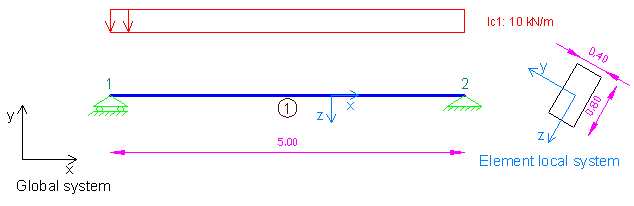# Example 2: Element local coordinate system (skew member) using 3D Frame Analysis Library

## Example 2: Element local coordinate system (skew member) using 3D Frame Analysis Library

In this example, we will call the 3D Frame Library from a Visual Studio project and carry out the structural analysis of a simple beam with skew cross section according to its local axis definition. We will first provide the geometry, material properties and loads and afterwards call the corresponding routine in order to obtain the results.Sample beam with local axis definition to analyze with 3D Frame Library (Metric units)

We will first create a new C# Windows Application project from Visual Studio and follow the following steps to carry out the structural analysis according the data provided. Please notice that we could use any other .NET compatible language and take the corresponding steps. The source code of all examples can be downloaded here.

## Add a reference to 3D Frame Library

A reference to Frame 3D Library can easily be added by right clicking on the application project and selecting Add --> Reference.

## Providing the data to 3D Frame Library using C#

### New model definition

``````Model Model = new Model();

### Definition of materials

``````//Create a new material for concrete
Material matConcrete = new Material();
matConcrete.Name = "Concrete";//Material name
matConcrete.Density = 2.5;//density in mass units/m3, for example tn/m3
matConcrete.G = 11538461;//shear modulus
matConcrete.E = 30000000;//elasticity modulus``````

### Definition of cross section

``````//Create a new beam section of dimensions 40cmx80xm
FrameElementSection secBeam40_80 = new FrameElementSection();
secBeam40_80.Name = "Beam40/80";//section name
secBeam40_80.A = 0.4 * 0.8;//section area
secBeam40_80.Iy = 0.4 * 0.8 * 0.8 * 0.8 / 12;//inertia moment about local y axis
secBeam40_80.Iz = 0.8 * 0.4 * 0.4 * 0.4 / 12;//inertia moment about local z axis
secBeam40_80.It = 0.0117248;//torsional constant
secBeam40_80.b = 0.40;//section height
secBeam40_80.h = 0.80;//section height``````

### Definition of model geometry and loads

``````//Create node n1
Frame3D.SuperNode n1 = new Frame3D.SuperNode(1, 0, 0, 0);
n1.dof1constraint = true;//translational constraint in direction y at local system of node
n1.dof2constraint = true;//translational constraint in direction y at local system of node
n1.dof3constraint = true;//translational constraint in direction z at local system of node
n1.dof4constraint = true;//rotational constraint in direction x at local system of node
n1.dof5constraint = true;//rotational constraint in direction y at local system of node
n1.dof6constraint = true;//rotational constraint in direction y at local system of node

//Create node n2
Frame3D.SuperNode n2 = new Frame3D.SuperNode(2, 5, 0, 0);
n2.dof1constraint = true;//translational constraint in direction x at local system of node
n2.dof2constraint = true;//translational constraint in direction y at local system of node
n2.dof3constraint = true;//translational constraint in direction z at local system of node
n2.dof4constraint = true;//rotational constraint in direction x at local system of node
n2.dof5constraint = true;//rotational constraint in direction y at local system of node
n2.dof6constraint = true;//rotational constraint in direction y at local system of node

//Create frame element 1
//Note the definition of the auxiliary point: Geometry.XYZ(0, Math.Tan(30/180*Math.PI), 1)
//It shows that the frame will be inserted properly (rotated about its longitudinal axis)
FrameSuperElement el1 = new FrameSuperElement(1, n1, n2, Geometry.XYZ(0, Math.Tan(30/180*Math.PI), 1), matConcrete, secBeam40_80, new MemberReleases(), new MemberReleases(), false, false);

### Call the solution method

``Model.Solve();``

### Obtain the analysis results

``````//-------OBTAIN RESULTS-------
double[] Min, Max;

//Reactions
n1.GetReactionsForLoadCase("lc1", out Min, out Max, 0);
double[] n1_R_lc1 = Max;
n2.GetReactionsForLoadCase("lc1", out Min, out Max, 0);
double[] n2_R_lc1 = Max;

//Note that element forces are now different, shear force acts on both y and z directions in element local system
el1.GetInternalForcesForLoadCase(0, "lc1", out Min, out Max, 0);//Internal forces at the start of the member
double[] forces_along_member_left = Max;
el1.GetInternalForcesForLoadCase(2.5, "lc1", out Min, out Max, 0);//Internal forces at the middle of the member
double[] forces_along_member_middle = Max;
el1.GetInternalForcesForLoadCase(5, "lc1", out Min, out Max, 0);//Internal forces at the end of the member
double[] forces_along_member_right = Max;

el1.GetDisplacementsForLoadCase(0, "lc1", out Min, out Max, 0);//Internal displacements at the start of the member
double[] disps_along_member_left = Max;
el1.GetDisplacementsForLoadCase(2.5, "lc1", out Min, out Max, 0);//Internal displacements at the middle of the member
double[] disps_along_member_middle = Max;
el1.GetDisplacementsForLoadCase(5, "lc1", out Min, out Max, 0);//Internal displacements at the end of the member
double[] disps_along_member_right = Max;``````